# NCERT Solutions for Class 10 Maths Chapter 8 Introduction to Trigonometry Ex 8.4

These NCERT Solutions for Class 10 Maths Chapter 8 Introduction to Trigonometry Ex 8.4 Questions and Answers are prepared by our highly skilled subject experts.

## NCERT Solutions for Class 10 Maths Chapter 8 Introduction to Trigonometry Exercise 8.4Question 1.
Express the trigonometric ratios sin A, sec A and tan A in terms of cot A.
Solution:
We know thatQuestion 2.
Write all the other trigonometric ratios of ∠A in terms of sec A.
Solution:
We know that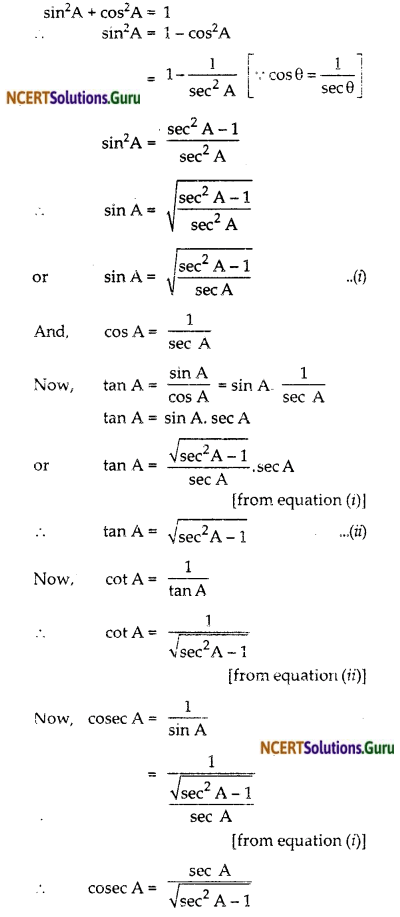Question 3.
Evaluate:
(i) $$\frac{\sin ^{2} 63^{\circ}+\sin ^{2} 27^{\circ}}{\cos ^{2} 17^{\circ}+\cos ^{2} 73^{\circ}}$$
(ii) sin 25° cos 65° + cos 25° sin 65°
Solution:
(i) We know that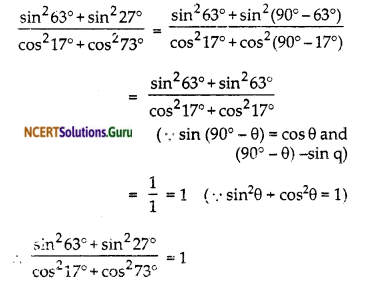(ii) We know that
sin 25° cos 65° + cos 25° sin 65°
= sin 25° cos (90° – 25°) + cos 25° sin (90° – 25°)
= sin 25° sin 25° + cos 25° cos 25°
= sin² 25° + cos² 25° = 1 (sin² θ + cos² θ)Question 4.
Choose the correct option. Justify your choice.
(i) 9 sec² A – 9 tan² A =
(A) 1
(B) 9
(C) 8
(D) 0

(ii) (1 + tan θ + sec θ) (1 + cot θ – cosec θ ) =
(A) 0
(B) 1
(C) 2
(D) -1

(iii) (sec A + tan A) (1 – sin A) =
(A) sec A
(B) sin A
(C) cosec A
(D) cos A

(iv) $$\frac{1+\tan ^{2} A}{1+\cot ^{2} A}$$
(A) sec² A
(B) -1
(C) cot² A
(D) tan² A
Solution:
(i) We know that
9 sec² A – 9 tan² A = 9(sec² A – tan² A)
= 9 x 1 = 9
Correct option is (B)

(ii) We know that
(1 + tan θ + sec θ) (1 + cot θ – cosec θ )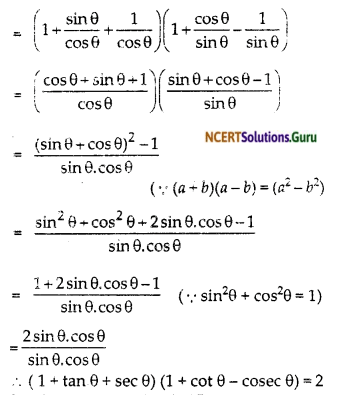So, the correct option is (C)

(iii) We have given,So, the correct option is (D)

(iv) We have given,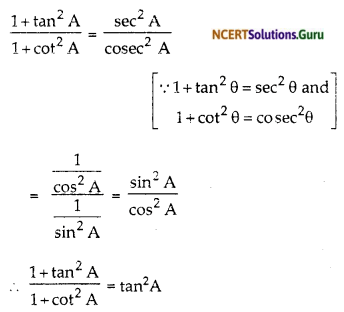So, the correct option is (D)Question 5.
Prove the following identities, where the angles involved are acute angles for which the expressions are defined.Solution:(vi) L.H.S
$$\sqrt{\frac{1+\sin A}{1-\sin A}}$$
Multiply both numerator and denominator by $$\sqrt{1+\sin A}$$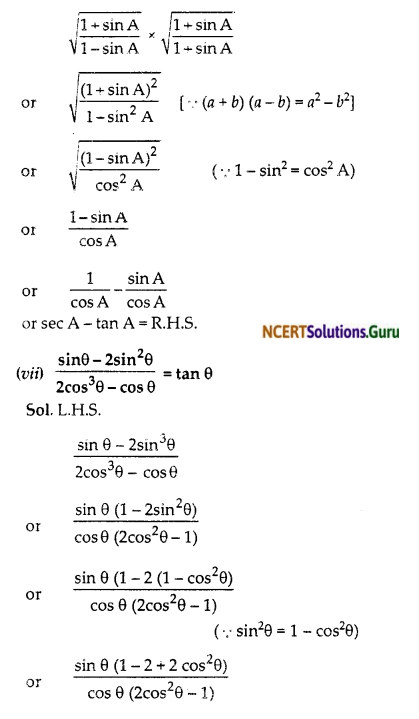(viii) (sin A + cosec A)² + (cos A + sec A)² = 7 + tan²A + cot²A.
Solution:
L.H.S.
(sin A + cosec A)² (cos A + sec A)²
= (sin² A + cosec² A + 2sin A + cosec A) + (cos²A + sec² A + 2cos. A sec A)
= sin² A + cosec² A + ²) + (cos² A + sec² A + 2) [∵sin A cosec A = 1 and cos A sec A = 1]
= (sin² A + cos² A) + 4 + sec² A + cosec² A
= 5 (1 + tan² A) + 1 + cot² A) [∵ sec² A = (1 + tan² A) and cosec² A = (1 + cot² A)]
= 7 tan² A + cot² A = R.H.S.

(ix) (cosec A – sin A) (sec A – cos A) = $$\frac{1}{\tan A+\cot A}$$
[Hint: Simplify LHS and RHS separately]
Solution:
L.H.S.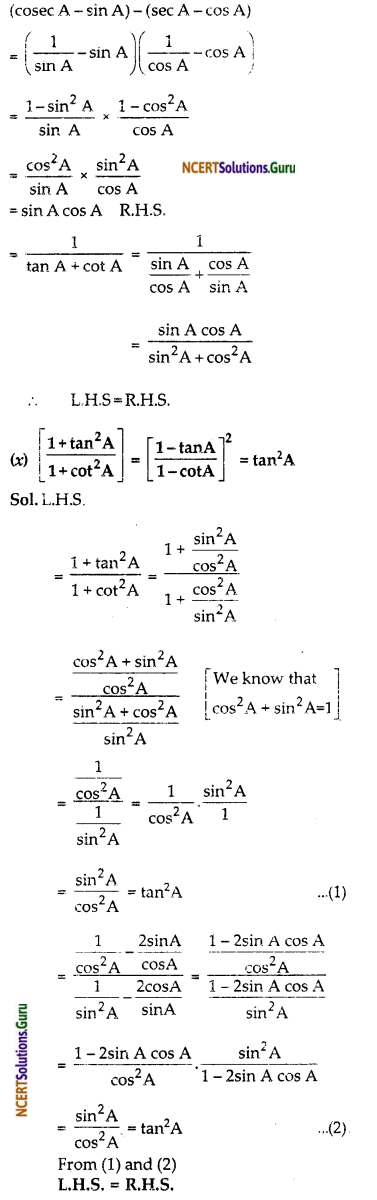error: Content is protected !!